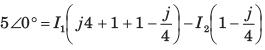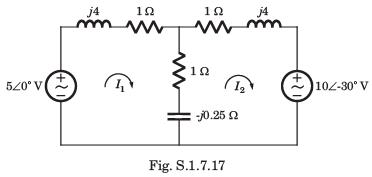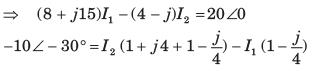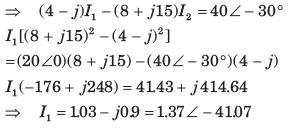Courses

# Test: Sinusoidal Steady‐state Analysis Part - 1

## 10 Questions MCQ Test Networking Theory | Test: Sinusoidal Steady‐state Analysis Part - 1

Description
This mock test of Test: Sinusoidal Steady‐state Analysis Part - 1 for Electrical Engineering (EE) helps you for every Electrical Engineering (EE) entrance exam. This contains 10 Multiple Choice Questions for Electrical Engineering (EE) Test: Sinusoidal Steady‐state Analysis Part - 1 (mcq) to study with solutions a complete question bank. The solved questions answers in this Test: Sinusoidal Steady‐state Analysis Part - 1 quiz give you a good mix of easy questions and tough questions. Electrical Engineering (EE) students definitely take this Test: Sinusoidal Steady‐state Analysis Part - 1 exercise for a better result in the exam. You can find other Test: Sinusoidal Steady‐state Analysis Part - 1 extra questions, long questions & short questions for Electrical Engineering (EE) on EduRev as well by searching above.
QUESTION: 1

### i(t) = ?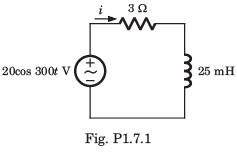Solution:

Z = 3 + j(25m)( 300) = 3 +  j7.5 Ω = 8.08     68.20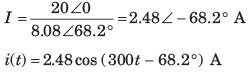QUESTION: 2

### vC(t) = ?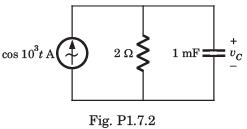Solution: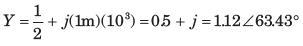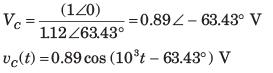QUESTION: 3

### vC(t) = ?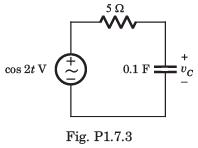Solution: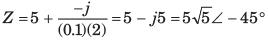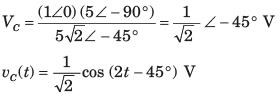QUESTION: 4

vC(t) = ?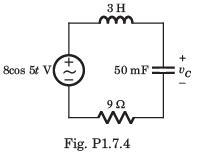Solution: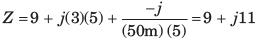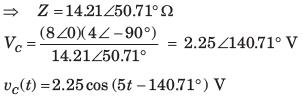QUESTION: 5

i(t) = ?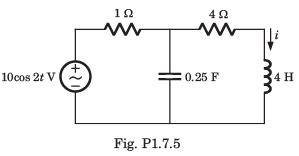Solution: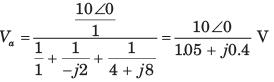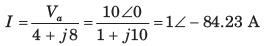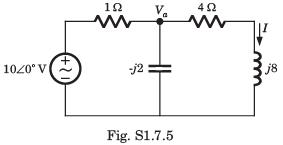i(t) = cos (2 t - 84.230) A

QUESTION: 6

In the bridge shown in fig. P1.7.13, Z1 = 300Ω, Z2= ( 300 - 600) Ω, Z3 = (200 + 100)Ω. The Z4 at balance is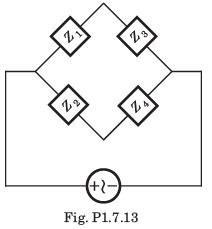Solution:

Z1Z4 = Z3Z2
300Z4 = (300-j600)(200+j100)
⇒ Z4 = 400 - j300

QUESTION: 7

In a two element series circuit, the applied voltage and the resulting current are v(t) = 60 + 66 sin (103t) V, i(t) = 2.3 sin (103t + 68.3.0) A. The nature of the elements would be

Solution:

R - C causes a positive phase shift in voltage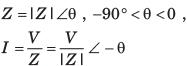QUESTION: 8

Vo = ?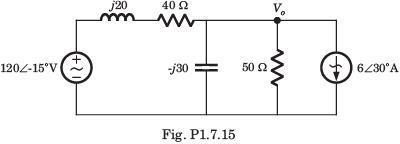Solution: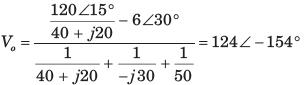QUESTION: 9

v0(t) = ?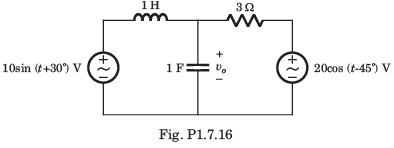Solution: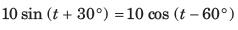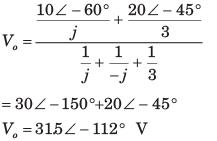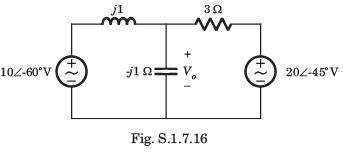QUESTION: 10

The circuit is as shown in fig. P1.7.17-18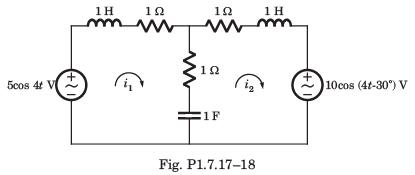Q. i1(t) = ?

Solution: